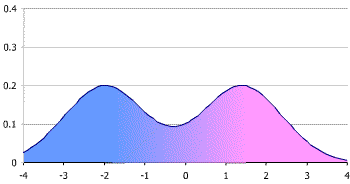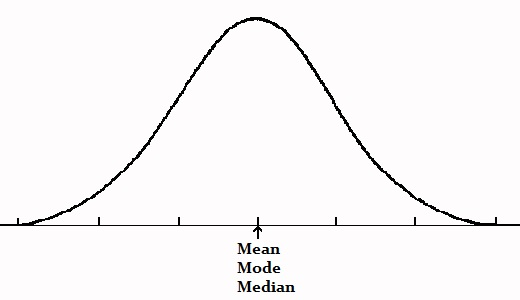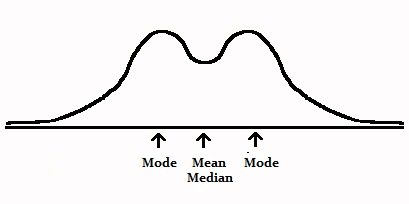# Symmetric Distribution in Statistics

Share on

Descriptive Statistics > Symmetric Distribution

A symmetric distribution is a type of distribution where the left side of the distribution mirrors the right side. By definition, a symmetric distribution is never a skewed distribution. This should be intuitive: try and draw lines of symmetry through a skewed distribution (you can’t).

The normal distribution is symmetric. It is also a unimodal distribution (it has one peak).

Distributions don’t have to be unimodal to be symmetric. They can be bimodal (two peaks) or multimodal (many peaks). The following bimodal distribution is symmetric, as the two halves are mirror images of each other.### Mean, Mode and Median in a Symmetric Distribution

In a symmetric distribution, the mean, mode and median all fall at the same point. The mode is the most common number and it matches with the highest peak (the “mode” here is different from the “mode” in bimodal or unimodal, which refers to the number of peaks).An exception is the bimodal distribution. The mean and median are still in the center, but there are two modes: one on each peak.### Other Symmetric Distributions

The normal distribution is the symmetric distribution you’re most likely to encounter in elementary statistics. However, there are other distributions that display symmetry:

## References

Fang, K. (2018). Symmetric Multivariate and Related Distributions. Chapman & Hall/CRC.

CITE THIS AS:
Stephanie Glen. "Symmetric Distribution in Statistics" From StatisticsHowTo.com: Elementary Statistics for the rest of us! https://www.statisticshowto.com/symmetric-distribution-2/
---------------------------------------------------------------------------Need help with a homework or test question? With Chegg Study, you can get step-by-step solutions to your questions from an expert in the field. Your first 30 minutes with a Chegg tutor is free!